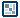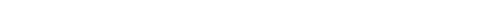## Question of the WeekFinding Values in an Array
by kirupa  |  4 September 2005

In Flash, you have the indexOf function that allows you to find text in an array of string data. That is great if you are only working with strings, but you will often run into situations where your array is more than just strings. If your array contains numbers, object references, etc., your indexOf function will not find a non-string value you search for.

So for the many cases that you need to scan through an array for a particular non-string data, the following function may come in handy:

contains = function (input, arrayData) {
for (i=0; i<arrayData.length; i++) {
if (arrayData[i] == input) {
return 1;
}
}
return -1;
};

How to Use It
Using the above function in your code is not complicated. Simply copy and paste the above function into the Actions window in Flash. With your code in place, you need an array that you will use to search through data for.

The function takes in two arguments, the keyword and the array. If your keyword is among the data found in the array, the function returns a 1. Otherwise, if the data can not be found, the function returns a -1.

Ideally, you would use the above function in conjunction with a conditional statement such as an if statement in the following example where I search for "kirupa" in an array called dataValues:

if(contains("kirupa", dataValues)==1) {
trace("Kirupa is found!");
}

Usage Examples
Copy and paste the following array and test cases into your Actions window after your contains function:

dataValues = ["kirupa", 10, Math.PI, "all have nothing in common!"];
// Test 1
trace(contains("kirupa", dataValues));
// Test 2
trace(contains("10", dataValues));
// Test 3
trace(contains(10, dataValues));
// Test 4
trace(contains(Math.PI, dataValues));
// Test 5
trace(contains("common", dataValues));

Press Ctrl + Enter. Notice the output of your various test cases. All of your tests should return a 1 (value found) with the exception of Test 5 that returns a -1 (value not found).

There are a few things that are interesting about the results. Notice that Tests 2 and 3 both return a 1, but 10 is a number in Test 2 but a string value in Test 3. The reason for that is because our contains function does not do type-checking. Our == operator in the for loop doesn't differentiate between a number and a string of the same number.

The lack of differentiation does not hold true for strings and variables though. Checking for the string "kirupa" and the variable kirupa will not equate. Flash will try to find what the variable kirupa is linked to as opposed to assuming that kirupa is equal to the string "kirupa".

Why the Code Works
Our contains function takes in two arguments, the keyword and the array, and does two things:

1. It cycles through values in your array.
2. It checks to see if the value you are currently on is the same as the keyword you are searching for.

The cycling is accomplished with a for loop that goes from index position 0 up to the total length of your array:

for (i=0; i<arrayData.length; i++) {
if (arrayData[i] == input) {
return 1;
}
}

For each iteration of your for loop, the variable i, increments accordingly. Since i is our index position, I can access the data from our array with the following command: arrayData[i]. If that value equals our keyword, then I return a 1 to indicate that the keyword has been found in our array:

for (i=0; i<arrayData.length; i++) {
if (arrayData[i] == input) {
return 1;
}
}

If after cycling through our array the value has not been found, I return a -1 to simulate a failed search return value similar to Flash's indexOf function.

Got a question or just want to chat? Comment below or drop by our forums (they are actually the same thing!) where a bunch of the friendliest people you'll ever run into will be happy to help you out!